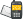It is currently 24 Nov 2020, 13:17### GMAT Club Daily Prep

#### Thank you for using the timer - this advanced tool can estimate your performance and suggest more practice questions. We have subscribed you to Daily Prep Questions via email.

Customized
for You

we will pick new questions that match your level based on your Timer History

Track

every week, we’ll send you an estimated GMAT score based on your performance

Practice
Pays

we will pick new questions that match your level based on your Timer History

#### Not interested in getting valuable practice questions and articles delivered to your email? No problem, unsubscribe here.# 100 tiles are labeled with the integers from 1 to 100 inclusQuestion banks Downloads My Bookmarks Reviews Important topics
Author Message
TAGS:
Retired ModeratorJoined: 07 Jun 2014
Posts: 4803
GRE 1: Q167 V156WE: Business Development (Energy and Utilities)
Followers: 175

Kudos [?]: 3033 , given: 394

100 tiles are labeled with the integers from 1 to 100 inclus [#permalink]
Expert's post00:00

Question Stats:86% (01:04) correct14% (00:47) wrongbased on 50 sessions
100 tiles are labeled with the integers from 1 to 100 inclusive; no numbers are repeated. If Alma chooses one tile at random, replaces it in the group, and chooses another tile at random, what is the probability that the product of the two integer values on the tiles is odd?

(A) $$\frac{1}{8}$$
(B) $$\frac{1}{4}$$
(C) $$\frac{1}{3}$$
(D) $$\frac{1}{2}$$
(E) $$\frac{3}{4}$$
[Reveal] Spoiler: OA

_________________

Sandy
If you found this post useful, please let me know by pressing the Kudos Button

Try our free Online GRE Test

ModeratorJoined: 07 Jan 2018
Posts: 697
Followers: 11

Kudos [?]: 785 , given: 88

Re: 100 tiles are labeled with the integers from 1 to 100 inclus [#permalink]
Total number of tiles = 100
Numbering starts with odd and ends with even. i.e from 1 to 100. Therefore there are 50 odd tiles and 50 even tiles.

For product of two number to be odd, both number has to be odd.
Hence number of successful outcome = 50 * 50
Number of possible outcome = 100* 100
Probability = 50*50/100*100 = 1/4

Posted from my mobile device_________________

Need Practice? 20 Free GRE Quant Tests available for free with 20 Kudos

Retired ModeratorJoined: 07 Jun 2014
Posts: 4803
GRE 1: Q167 V156WE: Business Development (Energy and Utilities)
Followers: 175

Kudos [?]: 3033 , given: 394

Re: 100 tiles are labeled with the integers from 1 to 100 inclus [#permalink]
Expert's post
Explanation

Use both probability and number properties concepts in order to answer this question.

First, in order for two integers to produce an odd integer, the two starting integers must be odd. An odd times an odd equals an odd. An even times an odd, by contrast, produces an even, as does an even times an even.

Within the set of tiles, there are 50 even numbers (2, 4, 6, …, 100) and 50 odd numbers (1, 3, 5, …,99).

One randomly-chosen tile will have a $$\frac{50}{100}=\frac{1}{2}$$ probability of being even, and a $$\frac{1}{2}$$ probability of being odd.

The probability of choosing an odd tile first is $$\frac{1}{2}$$ and the probability of choosing an odd tile second is also , so the probability of “first odd and second odd” is $$\frac{1}{2}\times\frac{1}{2}=\frac{1}{4}$$.
_________________

Sandy
If you found this post useful, please let me know by pressing the Kudos Button

Try our free Online GRE Test

GRE InstructorJoined: 10 Apr 2015
Posts: 3907
Followers: 163

Kudos [?]: 4766 , given: 70

Re: 100 tiles are labeled with the integers from 1 to 100 inclus [#permalink]
Expert's post
sandy wrote:
100 tiles are labeled with the integers from 1 to 100 inclusive; no numbers are repeated. If Alma chooses one tile at random, replaces it in the group, and chooses another tile at random, what is the probability that the product of the two integer values on the tiles is odd?

(A) $$\frac{1}{8}$$
(B) $$\frac{1}{4}$$
(C) $$\frac{1}{3}$$
(D) $$\frac{1}{2}$$
(E) $$\frac{3}{4}$$

There are 100 tiles. 50 tiles are EVEN and 50 tiles are ODD
The only way to get an ODD product is for the 1st tile to be ODD AND the 2nd tile to be ODD

So, P(product is ODD) = P(1st tile is ODD AND the 2nd tile is ODD)
= P(1st tile is ODD) x P(2nd tile is ODD)
= 50/100 x 50/100
= 1/2 x 1/2
= 1/4

Cheers,
Brent
_________________

Brent Hanneson – Creator of greenlighttestprep.comRe: 100 tiles are labeled with the integers from 1 to 100 inclus   [#permalink] 10 Aug 2018, 05:12
Display posts from previous: Sort by

# 100 tiles are labeled with the integers from 1 to 100 inclusQuestion banks Downloads My Bookmarks Reviews Important topicsPowered by phpBB © phpBB Group Kindly note that the GRE® test is a registered trademark of the Educational Testing Service®, and this site has neither been reviewed nor endorsed by ETS®.